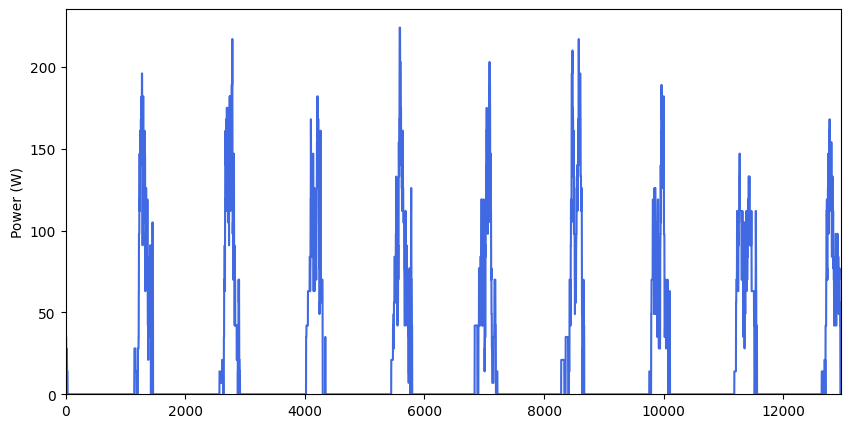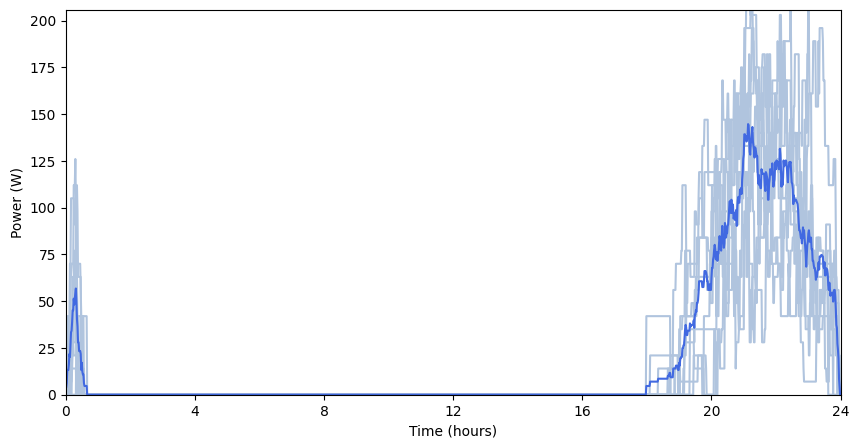# Appliances with occasional use¶

There are some appliances that are occasionally included in the mix pf appliances that the user switches-on during the day. For example, iron, stereo, printers and …

Within ramp, the user may specify the probability of using an appliance on the daily mix with a parameter called, occasional_use.

When occasional_use = 0, the appliance is always present in the mix and when occasional_use = 1, the appliance is never present.

The following example, investigates the effect of this parameter by modelling two user category: * A household that uses a computer with ocationally * A school that uses the computer everyday

```# importing functions
from ramp import User,calc_peak_time_range,yearly_pattern
import pandas as pd
```

## Creating a user category and appliances¶

```household = User("Household")
school = User("School")
```
```computer_0 = household.add_appliance(
name = "Household Computer",
number = 1,
power  = 50,
num_windows = 1,
func_time = 210,
occasional_use = 0.5,  # 50% chance of occasional use,
window_1 = [510,750],
)
```
```computer_1 = school.add_appliance(
name = "School Computer",
number = 1,
power  = 50,
num_windows = 1,
func_time = 210,
time_fraction_random_variability = 0.2,
func_cycle = 10,
occasional_use = 1, # always present in the mix of appliances,
window_1 = [510,750],
)
```
```# Checking the maximum profile of the two appliances

max_profile_c1 = pd.DataFrame(computer_0.maximum_profile,columns=[computer_0.name])
max_profile_c2 = pd.DataFrame(computer_1.maximum_profile,columns=[computer_1.name])

max_profile_c1.plot()
max_profile_c2.plot()
```
```<AxesSubplot:>
```## Generating profiles¶

```peak_time_range = calc_peak_time_range(
user_list = [household,school]
)
year_behaviour = yearly_pattern()
```
```# plotting profiles for 5 days
days = range(10)

for day in days:
prof_i = day,
peak_time_range = peak_time_range,
Year_behaviour = year_behaviour
)

prof_i = day,
peak_time_range = peak_time_range,
Year_behaviour = year_behaviour
)

pd.DataFrame(
data = [household_profile,school_profile],
columns = range(1440),
index = [household.user_name,school.user_name]
).T.plot(title=f"day - {day}")
```As it can be seen from the figures, the computer is always present in the school appliance mix while for household, is occasionally present.